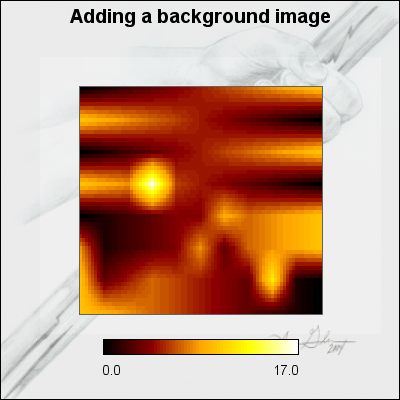## 22.5. Adding icon and text objects to the graph

Matrix plots supports the ordinary way of adding icon and text objects to the graph.

### 22.5.1. Adding a text object

Text objects are added by first creating an instance of `class Text` for each text needed and then adding the text to the graph with the usual call to `MatrixGraph::Add()`.

A basic text will only require two additional lines of code

 ```1 2 3 4 ``` ```Add(\$txt); ?>```

The following code snippet is slightly more complicated and will create a boxed text in the upper right corner of the graph.

 ```1 2 3 4 5 6 7 8 9 10 11 ``` ```SetFont(FF_ARIAL,FS_NORMAL,10); \$txt->Set("Arbitrary text\non a\nMatrix Plot"); \$txt->SetParagraphAlign('center'); \$txt->SetPos(0.95,0.15,'right'); \$txt->SetBox('lightyellow'); \$txt->SetShadow(); \$graph->Add(\$txt); ?>```

The snippet above adds a text at coordinates X=20, Y=20 using the default lower left corner as the text anchor point.

### Note

To add a newline you must remember to use double-quotes to enclose the text otherwise the "\n" will only be interpreted literally.

### Note

Remember that the "text align", as adjusted with `SetAlign()`, specifies the anchor point for the text, i.e. what part of the text is aligned with the specified position.

To add many text strings it is often useful to specify them in an array and then have a loop creating the text object and add the text array of all the created objects to the graph as the following short snippet shows.

 ```1 2 3 4 5 6 7 8 9 10 11 12 13 14 15 16 17 18 19 ``` ```SetFont(FF_ARIAL,FS_NORMAL,12); \$t[\$i]->SetColor('brown'); \$t[\$i]->SetAlign('center','top'); } \$graph->Add(\$t); ?>```

### 22.5.2. Adding icons to the graph

Icons are added as instances of `class IconPlot` to the graph (as usual with a call to `MatrixGraph::Add()`). This means that to use icons the library module "`jpgraph_iconplot.php`" must first be included.

The following example shows how to add a small icon in the lower right corner of the graph. For more information on formatting icons that are added to a graph see Section 14.14

### Caution

Since Matrix graphs doesn't have a ny concept of linear scale the position of icons can only be specified as absolute pixels or as fractions of the width/height.

Example 22.2. Adding an icon to the lower right corner (`matrix_ex03.php`)

 ```1 2 3 4 5 6 7 8 9 10 11 12 13 14 15 16 17 18 19 20 21 22 23 24 25 26 27 28 29 30 31 32 33 34 35 36 ``` ```title->Set('Adding an icon to the background'); \$graph->title->SetFont(FF_ARIAL,FS_BOLD,14); \$mp = new MatrixPlot(\$data,1); \$mp->SetSize(0.6); \$icon = new IconPlot('icon.jpg',\$width-1,\$height-1,0.8,50); \$icon->SetAnchor('right','bottom'); \$graph->Add(\$icon); \$graph->Add(\$mp); \$graph->Stroke(); ?>```

Figure 22.9. Adding an icon to the lower right corner `(matrix_ex03.php)`We also recall that it is possible to use country flags as icons by making use of the method

• `IconPlot::SetCountryFlag(\$aFlag,\$aX=0,\$aY=0,\$aScale=1.0,\$aMix=100,\$aStdSize=3)`

In just the same way as for all other plots it is possible to add a background images, background gradients and a background flag to the graph. Since this has been discussed several time previously in the manual we only show a simple example here.

### Note

Since Matrix graphs does not have the concept of plot area the background image positioning alternative `BGIMG_FILLPLOT` is the same as `BGIMG_FILLFRAME` as we have used in this example below.

Example 22.3. Adding a background image to the matrix graph (`matrix_ex04.php`)

 ```1 2 3 4 5 6 7 8 9 10 11 12 13 14 15 16 17 18 19 20 21 22 23 24 25 26 27 28 29 30 31 32 33 34 35 36 37 38 ``` ```title->Set('Adding a background image'); \$graph->title->SetFont(FF_ARIAL,FS_BOLD,14); // Add a stretched background image \$graph->SetBackgroundImage('ironrod.jpg',BGIMG_FILLFRAME); \$graph->SetBackgroundImageMix(50); \$mp = new MatrixPlot(\$data,1); \$mp->SetSize(0.6); \$mp->SetCenterPos(0.5,0.5); \$mp->SetLegendLayout(1); \$graph->Add(\$mp); \$graph->Stroke(); ?>```

Figure 22.10. Adding a background image to the matrix graph `(matrix_ex04.php)`